Home > CC4 > Chapter 11 > Lesson 11.2.2 > Problem11-67

11-67.
1. Solve each equation below. Then check your solutions. Explain your steps for part (a). Homework Help ✎

2.  a.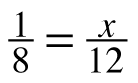b.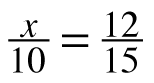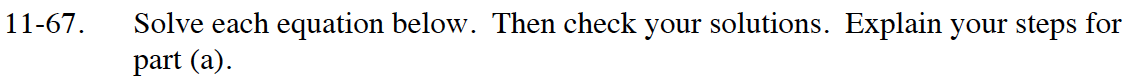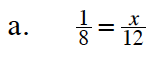Review the Math Notes box in section 3.3.2

A good fraction buster number would be 24.
Multiply both sides of the equation by 24 to clear the fraction.
Now complete the problem and remember to explain your steps.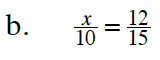x = 8